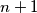IMO Shortlist 2014 problem C1

Kvaliteta:
Avg: 0,0
Težina:
Avg: 6,0

Let$n$ points be given inside a rectangle$R$ such that no two of them lie on a line parallel to one of the sides of$R$. The rectangle$R$ is to be dissected into smaller rectangles with sides parallel to the sides of$R$ in such a way that none of these rectangles contains any of the given points in its interior. Prove that we have to dissect$R$ into at least$n + 1$ smaller rectangles.

(Serbia)

Izvor: https://www.imo-official.org/problems/IMO2014SL.pdf